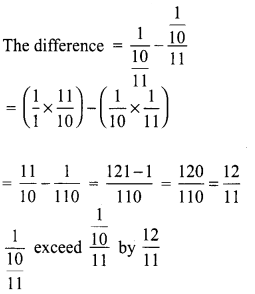Students can Download Maths Chapter 1 Rational Numbers Ex 1.3 Questions and Answers, Notes Pdf, Samacheer Kalvi 8th Maths Book Solutions Guide Pdf helps you to revise the complete Tamilnadu State Board New Syllabus and score more marks in your examinations.## Tamilnadu Samacheer Kalvi 8th Maths Solutions Term 1 Chapter 1 Rational Numbers Ex 1.3

Question 1.
Match the following appropriately.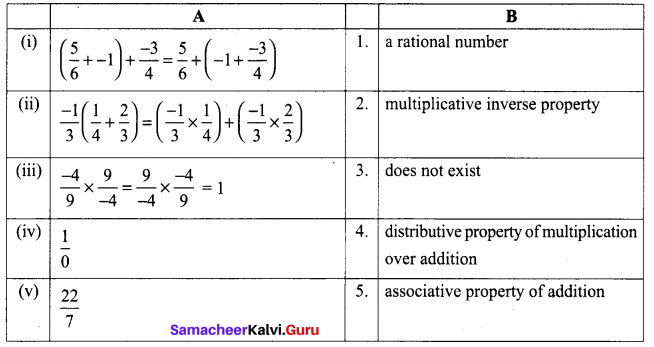Solution:
(i) 5
(ii) 4
(iii) 2
(iv) 3
(v) 1

Question 2.
Which of the following properties hold for subtraction of rational numbers? Why?
(a) closure
(b) commutative
(c) associative
(d) identity
(e) inverse
Solution:
(i) For subtraction of rational numbers closure property is true.
Because for any two rational number a and b, a + b is in Q.
Eg. $$-\frac{1}{4}+\frac{3}{2}=\frac{-1+6}{4}=\frac{5}{4}$$ is rational.
(ii) Commutative fails as $$\frac{1}{3}-\frac{2}{4} \neq \frac{2}{4}-\frac{1}{3}$$
(iii) Associative fails as $$\frac{1}{2}-\left(\frac{1}{3}-\frac{1}{4}\right) \neq\left(\frac{1}{2}-\frac{1}{3}\right)-\frac{1}{4}$$
(iv) Identity fails as 5 – 0 ≠ 0 – 5
(v) Inverse also fails.

Question 3.
Subbu spends $$\frac{1}{3}$$ of his monthly earnings on rent, $$\frac{2}{5}$$ on food and $$\frac{1}{10}$$ on monthly usuals. What fractional part of his earnings is left with him for other expenses?
Solution: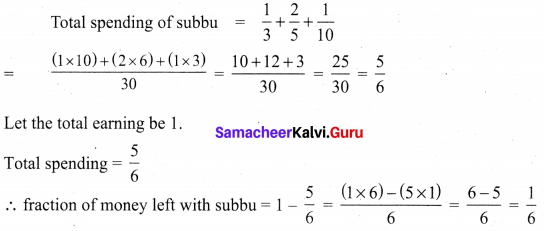Question 4.
In a constituency, $$\frac{19}{25}$$ of the voters had voted for candidate A whereas $$\frac{7}{50}$$ had voted for candidate B. Find the fraction of the voters who had voted for other.
Solution: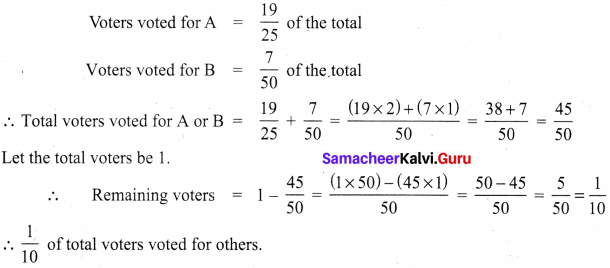Question 5.
If $$\frac{3}{4}$$ of a box of apples weighs 3 kg and 225 gm, how much does a full box of apples weigh?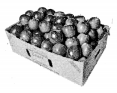Solution:
Let the total weight of a box of apple = x kg.
Weight of $$\frac{3}{4}$$ of a box apples = 3 kg 225 gm. = 3.225 kg
$$\frac{3}{4}$$ × x = 3225
x = $$\frac{3.225 \times 4}{3}$$ kg
= 1.075 × 4 kg = 4.3 kg = 4 kg 300 gm
Weight of the box of apples = 4 kg 300 gm.

Question 6.
Mangalam buys a water jug of capacity 3$$\frac{4}{5}$$ litres. If she buys another jug which is 2$$\frac{2}{3}$$ times as large as the smaller jug, how many litres can the larger one hold?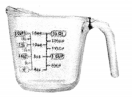Solution: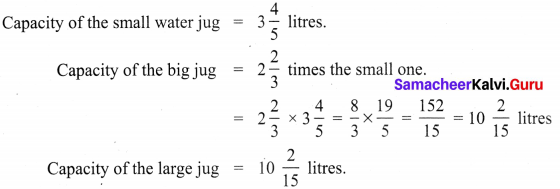Question 7.
In a recipe making, every $$1 \frac{1}{2}$$ cup of rice requires $$2 \frac{3}{4}$$ cups of water. Express this in the ratio of rice to water.
Solution:
For the recipe rice required = $$1 \frac{1}{2}$$ cup; water required = $$2 \frac{3}{4}$$ cups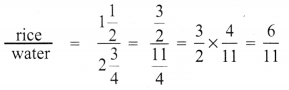∴ rice : water = 6 : 11

Question 8.
Ravi multiplied $$\frac{25}{8}$$ and $$\frac{16}{15}$$ to obtain $$\frac{400}{120}$$. He says that the simplest form of this product is $$\frac{10}{3}$$ and Chandru says the answer in the simplest form is $$3 \frac{1}{3}$$. Who is correct? or Are they both correct? Explain.
Solution: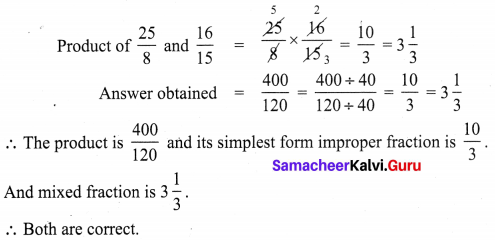Question 9.
A piece of wire is $$\frac{4}{5}$$ m long. If it is cut into 8 pieces of equal length, how long will each piece be?
Solution:
Length of the wire = $$\frac{4}{5}$$ m = $$\frac{4 \times 100}{5}$$ cm = 80 cm
Number of equal pieces made from it = 8 Length of a single piece = 80 ÷ 8 = 10 cm
Length of each small pieces = 10 cm.Question 10.
Find the length of a room whose area is $$\frac{153}{10}$$ sq.m and whose breadth is $$2 \frac{11}{20}$$ m.
Solution:
Breadth of the room = $$2\frac{11}{20}$$ m; Area of the room = $$\frac{153}{10}$$ sq.m
Length of the room × Breadth = Area of the room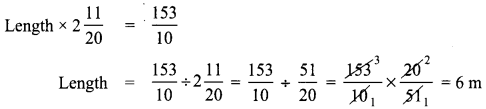Length of the room = 6 m

Challenging Problems

Question 1.
Show that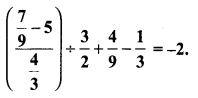Solution: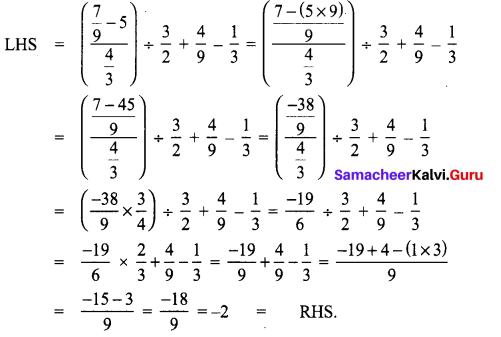Question 2.
If A walks $$\frac{7}{4}$$ km and then jogs $$\frac{3}{5}$$ km, find the total distance covered by A. How much did A walk rather than jog?
Solution:
Distance walked by A = $$\frac{7}{4}$$ km; Distance jogged by A = $$\frac{3}{5}$$ km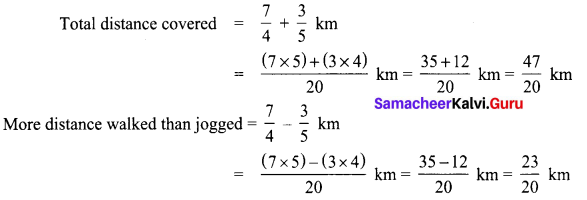Question 3.
In a map, if 1 inch refers to 120km, then find the distance between two cities B and C which are $$4 \frac{1}{6}$$ inches and $$3 \frac{1}{3}$$ inches from the city A which is in between the cities B and C.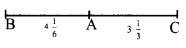Solution:
1 inch = 120 km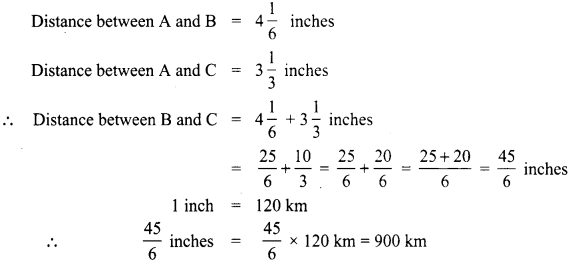Distance between B and C = 900 km

Question 4.
Give an example for each of the following statements.
(i) The collection of all non-zero rational numbers is closed under division.
(ii) Subtraction is not commutative for rational numbers.
(iii) Division is not associative for rational numbers.
(iv) Distributive of multiplication over subtraction is true for rational numbers, that is a (b – c) = ab – ac.
(v) The mean of two rational numbers is rational and lies between them.
Solution:
(i) Let a = $$\frac{5}{4}$$ and b = $$\frac{-4}{3}$$ be two non zero rational numbers.
a ÷ b = $$\frac{5}{6} \div \frac{-4}{3}=\frac{5}{6} \times \frac{3}{-4}=\frac{5}{-8}$$ is in Q
∴ Collection of non-zero rational numbers are closed under division.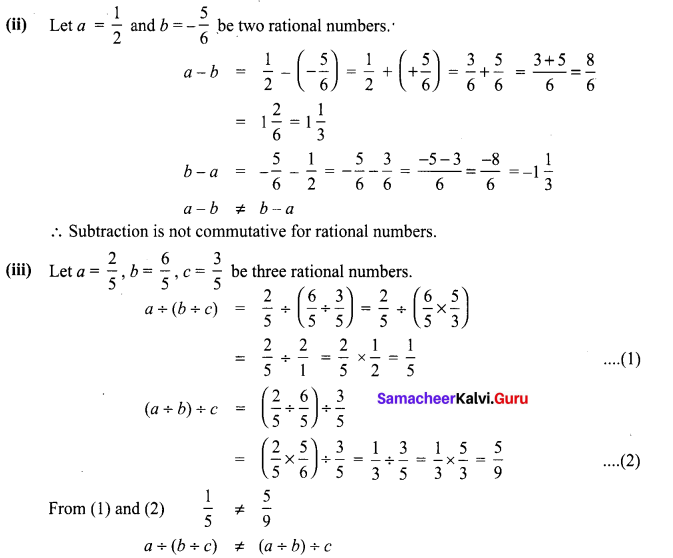∴ Division is not associative for rational numbers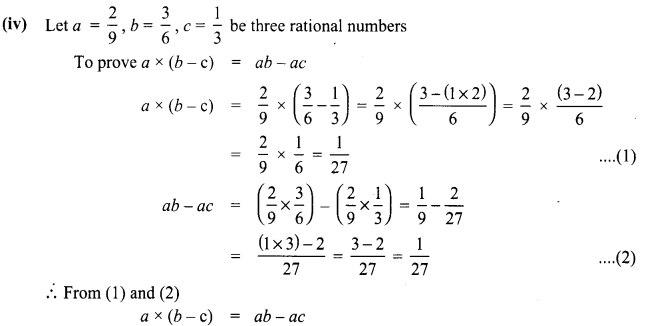∴ From (1) and (2)
a × (b – c) = ab – bc
∴ Distributivity of multiplication over subtraction is true for rational numbers.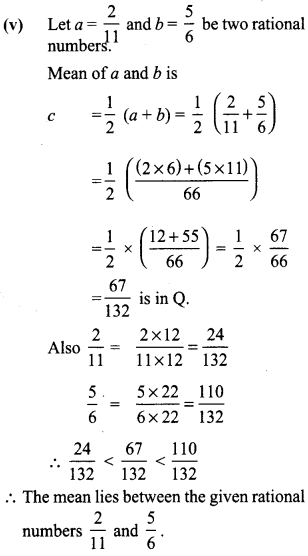Question 5.
If $$\frac{1}{4}$$ of a ragi adai weighs 120 grams, 4what will be the weight of $$\frac{2}{3}$$ of the same ragi adai?
Solution:
Let the weight of 1 ragi adai = x grams given $$\frac{1}{4}$$ of x = 120 gm
$$\frac{1}{4}$$ × x = 120
x = 120 × 4
x = 480 gm
∴ $$\frac{2}{3}$$ of the adai
= $$\frac{2}{3}$$ × 480 gm = 2 × 160 gm = 320 gm
$$\frac{2}{3}$$ of the weight of adai = 320 gm

Question 6.
Find the difference between the greatest and the smallest of the following rational numbers.
$$\frac{-7}{12}, \frac{2}{-9}, \frac{-11}{36}, \frac{-5}{-6}$$
Solution:
Here $$\frac{-5}{-6}=\frac{5}{6}$$ and is a positive rational number.
All other numbers are negative numbers
∴ $$\frac{-5}{-6}$$ is the greatest number
LCMof 12, 9, 36 = 3 × 4 × 3 = 36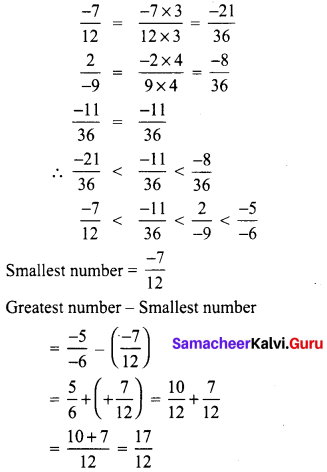Question 7.
If p + 2q = 18 and pq = 4o, find $$\frac{2}{p}+\frac{1}{q}$$
Solution:
Given p + 2q = 18 …………… (1)
pq = 40 ………… (2)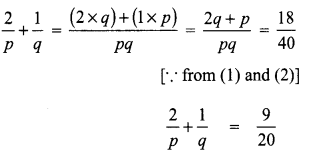Question 8.
Find ‘x’ $$5 \frac{x}{5} \times 3 \frac{3}{4}$$ = 21.
Solution: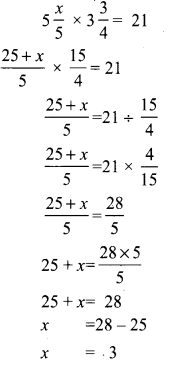Question 9.
The difference between a number and its two third is 30 more than one -fifth of the number. Find the numbers.
Solution:
Let the number to be find out = x
Its two third = $$\frac{2 x}{3}$$
Given x – $$\frac{2}{3}$$ x = $$\frac{1}{5}$$ x + 30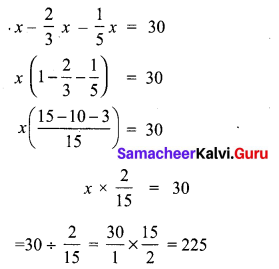Question 10.
By how much does $$\frac{1}{\frac{10}{11}}$$ exceed $$\frac{1}{\frac{10}{11}}$$?
Solution: